# WBJEE 2019 Maths Paper with Solutions

WBJEE 2019 Mathematics paper solved by the subject experts at BYJU’S is available on this page. The solutions have been provided in a detailed manner and the question paper along with answer key and solutions are in downloadable PDF form for offline viewing at a later stage. Candidates can gain a much clearer understanding of the questions, the paper pattern, weightage of marks as well as the concepts themselves by engaging in solving the question paper. This will help them study productively and prepare effectively for the entrance examination.

### WBJEE 2019 - Maths

Question 1: P is the extremity of the latus rectum of ellipse 3x2 + 4y2 = 48 in the first quadrant. The eccentric angle of P is

1. a. π/8
2. b. 3π/4
3. c. π/3
4. d. 3π/3

Solution:

Given

Equation of ellipse is

$$\frac{x^{2}}{16}+\frac{y^{2}}{12}=1$$

Let point P on curve (4cos θ,

$$2\sqrt{3}sin\theta$$
)

But we know point P is extremity of latus rectum of ellipse

So, P is (2, 3)

On comparing point P

4 cos θ= 2 and

$$2\sqrt{3}sin\theta$$
=3

Cos θ = (1/2) and sin θ = √3/2 ⇒ θ= π/3

Question 2: The direction ratios of the normal of the plane through the points (1,2,3), (–1,–2,1) and parallel to

$$\frac{x-2}{2}=\frac{y+1}{3}=\frac{z}{4}$$
is

1. a. (2, 3, 4)
2. b. (14, –8, –1)
3. c. (–2, 0, –3)
4. d. (1, –2, –3)

Solution:

Normal of the plane passing through the points (1, 2, 3), (–1, –2, 1) and parallel to given line is

=

$$\begin{vmatrix} \hat{i} &\hat{j} & \hat{k}\\ 2 & 3 & 4\\ 1+1 & 2+2 & -3-1 \end{vmatrix}=\begin{vmatrix} \hat{i} & \hat{j} & \hat{k}\\ 2 &3 & 4\\ 2 & 4 & -4 \end{vmatrix}$$

=

$$-28\hat{i}+16\hat{j}+2\hat{k}$$

Direction ratio’s of the normal to plane is < 14, –8, –1>

Question 3: The equation of the plane which bisects the line joining the points (1, 2, 3) and (3, 4, 5) at right angles is

1. a. x + y + z = 0
2. b. x + y – z = 9
3. c. x + y +z = 9
4. d. x + y – z + 9 = 0

Solution:

Equation of plane is (3 – 1)x +(4 – 2)y + (5 – 3)z = k …..(1)

This plane passes through m

$$=\left [ \frac{3+1}{2},\frac{2+4}{2},\frac{5+3}{2} \right ]$$

= (2, 3, 4)

⇒k = 2 × 2 + 2 × 3 + 2 × 4 = 18 (put in eq (1))

⇒ Eq. of plane is x + y + z =9

Question 4: The limit of the interior angle of a regular polygon of n sides as n → ∞is

1. a. π
2. b. π/8
3. c. 3π/2
4. d. 2π/3

Solution:

We know,

One interior angle = (n-2) π/n

$$\lim_{n\rightarrow \infty }\frac{(n-2)\pi }{n}=\pi$$

Question 5: Let f(x) > 0 for all x and f ‘(x)’ exists for all x. If f is the inverse function of h and

$$h'(x)=\frac{1}{1+log x}$$
. Then f ‘(x)’ will be

1. a. 1 + log (f(x))
2. b. 1+ f(x)
3. c. 1 – log (f(x))
4. d. logf(x)

Solution:

Given

⇒f(x) =h-1(x)

⇒h(f(x)) = x

On differentiating with respect to x

⇒h’(f(x)) . f’(x) = 1

⇒ f’(x) = [1/ h’(f(x))] = 1 + log (f(x))

Question 6: Consider the function f(x) = cosx2. Then

1. a. f is of period 2π
2. b. f is of period √2π
3. c. f is not periodic
4. d. f is of periodic π

Solution:

Given

f(x) = cosx2

Let, f(x) is periodic with period T

We know, f(x + T) = f(x)

⇒cos (x + T)2 = cos x2

⇒ cos (x + T)2 – cosx2 = 0

$$-2sin\left ( \frac{(x+T)^{2}-x^{2}}{2} \right )sin\left ( \frac{(x+T)^{2}+x^{2}}{2} \right )=0$$

⇒ (x + T)2 – x2 = nπ or (x + T)2 + x2 = nπ

Which is not possible because these equations are quadratic equation not identity

⇒ f(x) is not periodic

Question 7:

$$\lim_{x\rightarrow 0^{+}}(e^{x}+x)^{1/x}$$

1. a. Does or exist finitely
2. b. is 1
3. c. is e2
4. d. is 2

Solution:

$$\lim_{x\rightarrow 0^{+}}(e^{x}+x)^{1/x}(1^{\infty })$$

$$\lim_{e^{x\rightarrow 0^{+}}}\frac{(e^{x}+x)-1}{x}$$

Using binomial expansion of ex =1 + x + x2/2!+…….

⇒ e 1+1 =e2

Question 8: Let f(x) be a derivable function, f ‘(x) > f(x) and f(0) = 0. Then

1. a. f(x) > 0 for all x > 0
2. b. f(x) < 0 for all x > 0
3. c. no sign of f (x) can be ascertained
4. d. f(x) is a constant function

Solution:

Given

f ‘(x) > f(x)

Multiply e–x both sides

⇒f’(x). e–x – f(x).e–x> 0

⇒ (f(x). e–x)’> 0

⇒e –x. f(x) is increasing function

$$e^{-x}.f(x)> e^{-0}.f(0)\forall x> 0$$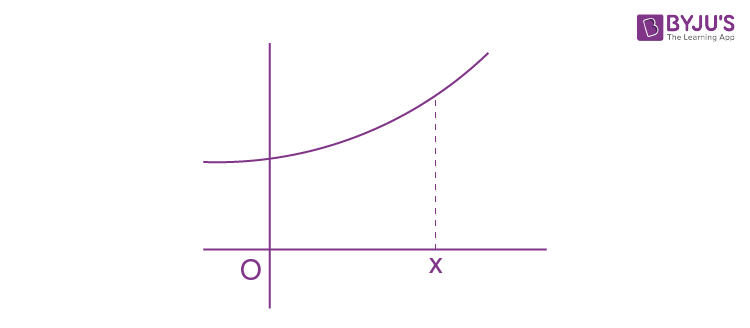⇒e –x. f(x) >0 since, f(0) = 0 (given)

$$f(x)> 0 \forall x> 0$$

Question 9: Let f: [1, 3] → R be a continuous function that is differentiable in (1, 3) and f ‘(x) = |f(x)|2 + 4 for all x ∈ (1, 3). Then

1. a. f(3) – f(1) = 5 is true
2. b. f(3) – f(1) = 5 is false
3. c. f(3) – f(1) = 7 is false
4. d. f(3) – f(1) < 0 only at one point (1, 3)

Solution:

f ‘(x) = |f(x)|2 + 4

Using LMVT theorem

$$\frac{f(3)-f(1)}{3-1}$$
= f’ (c) for atleast one c∈ (1, 3)

⇒f(3) – f(1) = 2(f(c))2 + 8

⇒f(3) – f(1) ≥ 8

⇒f(3) – f(1) = 5 (false)

Similarly f(3) – f(1) = 7 (false)

Question 10:

$$\lim_{x\rightarrow 0^{+}}(x^{n}lnx),n> 0$$

1. a. does not exist
2. b. exists and is zero
3. c. exists and is 1
4. d. exists and is e–1

Solution:

$$\lim_{x\rightarrow 0^{+}}(x^{n}lnx),n> 0$$

$$\lim_{x\rightarrow 0^{+}}\left [ \frac{lnx}{1/x^{n}} \right ]\left [ \frac{\infty }{\infty } \right ]$$
form

Using L-Hospital Rule

$$\lim_{x\rightarrow 0^{+}}\left [ \frac{1/x}{\frac{-n}{x^{n+1}}} \right ]$$

$$\lim_{x\rightarrow 0^{+}}\left [ \frac{x^{n}}{-n} \right ]=0$$

Question 11: If

$$\int \cos xlog\left(\tan \frac{x}{2}\right) d x=\sin x \log \left(\tan \frac{x}{2}\right)+f(x)$$
then f(x) is equal to, (assuming c is a arbitrary real constant)

1. a. c
2. b. c – x
3. c. c + x
4. d. 2x + c

Solution: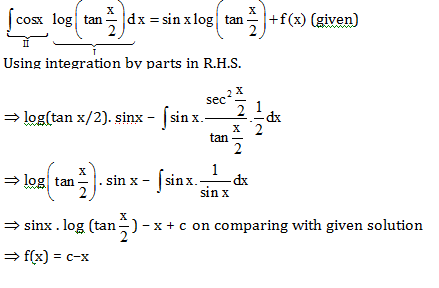Question 12:

$$y=\int \cos \left\{2 \tan ^{-1} \sqrt{\frac{1-x}{1+x}}\right\} dx$$
is an equation of a family of

1. a. straight lines
2. b. circles
3. c. ellipses
4. d. parabolas

Solution: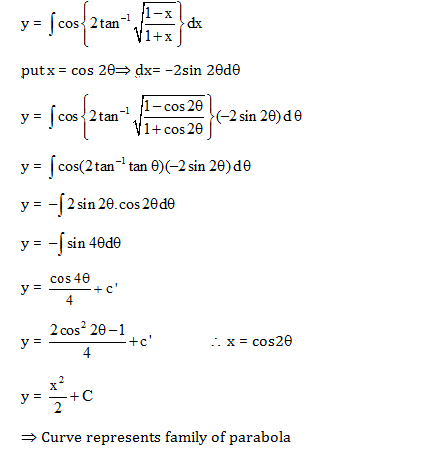Question 13: The value of the integration

$$\int_{-\pi / 4}^{\pi / 4}\left(\lambda|\sin x|+\frac{\mu \sin x}{1+\cos x}+\gamma\right) d x$$

1. a. is independent of λ only
2. b. is independent of μ only
3. c. in independent of γ only
4. d. depends on γ, μ and λ

Solution: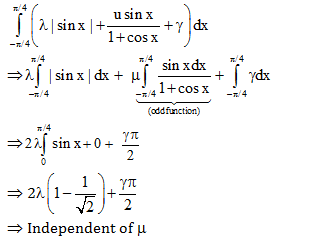Question 14: The value of

$$\lim _{x \rightarrow 0} \frac{1}{x}\left[\int_{y}^{a} e^{\sin ^{2} t} d t-\int_{x+y}^{a} e^{\sin ^{2} t} d t\right]$$
is equal to

1. a.
$$e^{sin^{2}y}$$
2. b. e2siny
3. c.
$$e^{\left | siny \right |}$$
4. d.
$$e^{cosec^{2}y}$$

Solution: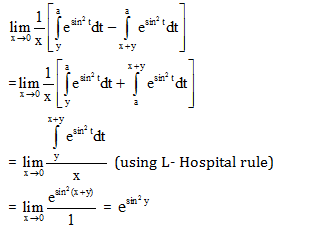Question 15: If

$$\int 2^{2^x}.2^{x}dx=A2^{2^x}+c$$
, then A =

1. a. 1/log x
2. b. log 2. c. (log2)2
3. d. 1/(log2)2

Solution:

$$\int 2^{2^x}.2^{x}dx=A2^{2^x}+c$$
---------(1)

Put 22x = t ⇒(22^x.ln2)(2x.ln2)dx = dt

$$\Rightarrow \int \frac{dt}{(ln2)^{2}}$$

$$\Rightarrow \frac{t}{(ln2)^{2}}+C\Rightarrow \frac{2^{2x}}{ln2^{2}}+C$$

(on comparing with equation (1))

⇒A = 1/(ln2)2

Question 16: The value of the integral

$$\int_{-1}^{1}\left\{\frac{x^{2015}}{e^{|x|}\left(x^{2}+\cos x\right)}+\frac{1}{e^{|x|}}\right\} d x$$
is equal to

1. a. 0
2. b. 1 – e–1
3. c. 2e–1
4. d. 2(1 – e–1)

Solution: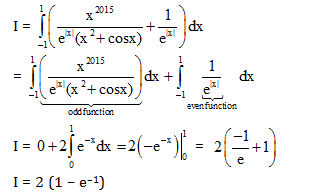Question 17:

$$\lim _{x \rightarrow \infty} \frac{1}{n}\left\{1+\sqrt{\frac{n}{n+3}}+\sqrt{\frac{n}{n+6}}+\sqrt{\frac{n}{n+9}}+\ldots+\sqrt{\frac{n}{n+3(n-1)}}\right\}$$

1. a. does not exist
2. b. is 1
3. c. is 2
4. d. is 3

Solution: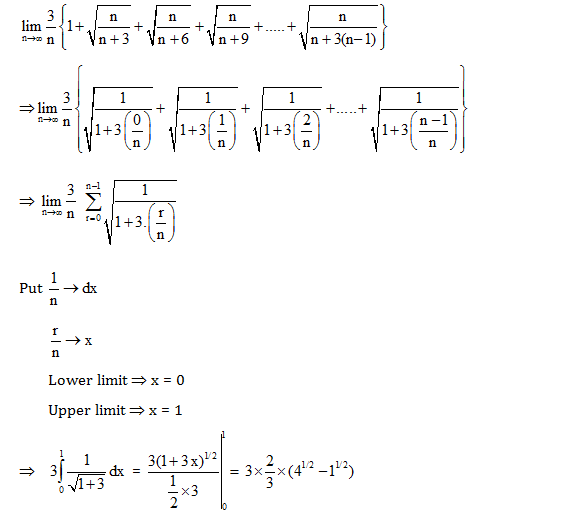= (2/3) x 3 =2

Question 18: The general solution of the differential equation

$$\left(1+e^{\frac{x}{y}}\right) d x+\left(1-\frac{x}{y}\right) e^{\frac{x}{y}} d y=0$$
is (c is an arbitrary constant)

1. a. x-ye(x/y) = c
2. b. y-xe(x/y) =c
3. c. x+ye(x/y)= c
4. d. y+xe(x/y) = c

Solution: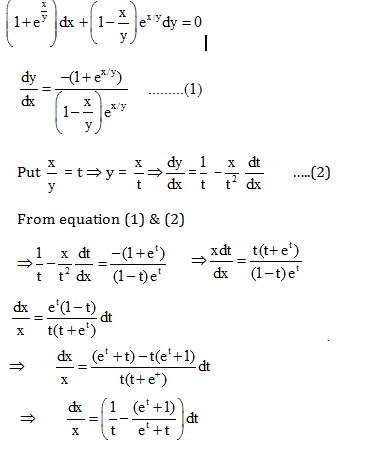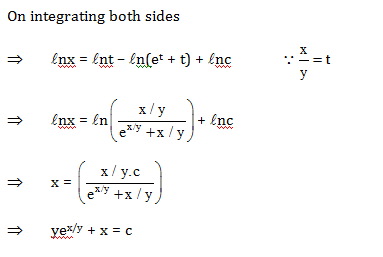Question 19: General solution of

$$\left ( x+y \right )^{2}\frac{dy}{dx}= a^{2}, a \neq 0$$
is (c is arbitrary constant)

1. a. (x/a) = tan(y/a) +c
2. b. tan xy = c
3. c. tan (x+y)= c
4. d. tan(y+c/a) = (x+y/a)

Solution:

$$(x+y)^{2}\frac{dy}{dx}=a^{2}\Rightarrow \frac{dy}{dx}=\frac{a^{2}}{(x+y)^{2}}$$

Put x + y = t ⇒ 1 + (dy/dx) = (dt/dx) …….(2)

⇒ From (1) & (2)

$$\Rightarrow dx=\frac{t^{2}}{t^{2}+a^{2}}dt$$

$$\Rightarrow dx=\left [ 1-\frac{a^{2}}{t^{2}+a^{2}} \right ]dt$$

Integrating both sides

⇒x + c = t – a tan–1 t/a since, t = x + y

⇒x = x + y – a tan–1(x+y/a) +c

$$\Rightarrow atan^{-1}\left ( \frac{x+y}{a} \right )=y+c\Rightarrow \frac{x+y}{a}=tan\left (\frac{y+c}{a} \right )$$

Question 20. Let P(4, 3) be a point on the hyperbola (x2/a2)-(y2/b2)=1.If the normal at P intersects the x-axis at (16, 0), then the eccentricity of the hyperbola is

1. a.
$$\frac{\sqrt{5}}{2}$$
2. b. 2
3. c.
$$\sqrt{2}$$
4. d.
$$\sqrt{3}$$

Solution: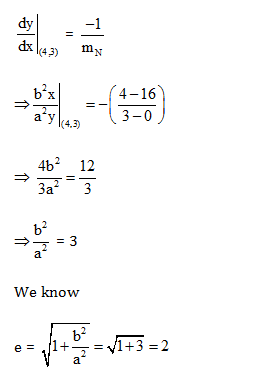Question 21: If the radius of a spherical balloon increases by 0.1% then its volume increases approximately by

1. a. 0.2%
2. b. 0.3%
3. c. 0.4%
4. d. 0.05%

Solution:

Volume of spherical balloon V = (4/3)πr3

\begin{aligned} \frac{\Delta \mathrm{V}}{\mathrm{V}} \times 100=\frac{\left(\frac{4}{3} \pi\left(\mathrm{r}+\frac{\mathrm{r}}{10}\right)^{3}-\frac{4}{3} \pi \mathrm{r}^{3}\right)}{\frac{4}{3} \pi^{3}} \times 100 \\ &=\left(1+\frac{1}{10}\right)^{3}-1 \end{aligned}

= 1 +(3/10) +(3/100) +(1/1000) –1 ≈ 0.3% approximately

Question 22: The three sides of a right-angled triangle are in G.P. (Geometrical Progression). If the two acute angles be α and β, then tan α and tan β are:

1. a.
$$\frac{\sqrt{5}+1}{2} and \frac{\sqrt{5}-1}{2}$$
2. b.
$$\sqrt{\frac{\sqrt{5}+1}{2}} and \sqrt{\frac{\sqrt{5}-1}{2}}$$
3. c.
$$\sqrt{5}and \frac{1}{\sqrt{5}}$$
4. d.
$$\frac{\sqrt{5}}{2}and \frac{2}{\sqrt{5}}$$

Solution:

Let sides are a, ar, ar2 (r > 1)

Using Pythagoras theorem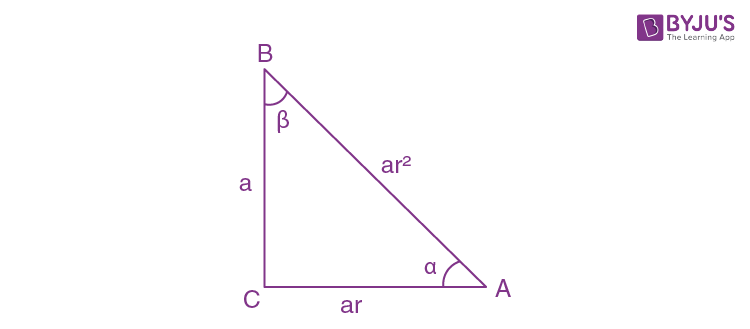⇒a2 + a2r2 = a2 r4

⇒r4 + r2–1 = 0

$$\Rightarrow r^{2}=\frac{-1+\sqrt{5}}{2},\frac{-1-\sqrt{5}}{2}$$
(not possible)

$$r=\sqrt{\frac{-1+\sqrt{5}}{2}}$$

$$tan\alpha =\frac{1}{r}$$

$$=\sqrt{\frac{2}{\sqrt{5}-1}}=\sqrt{\frac{\sqrt{5}+1}{2}}$$

$$tan\beta =r=\sqrt{\frac{\sqrt{5}-1}{2}}$$

Question 23: If

$$log_{2}6+\frac{1}{2x}=log_{2}\left [ 2^{1/x}+8 \right ]$$
, then the value of x are

1. a. (1/4,1/3)
2. b. (1/4 ,1/2)
3. c. (-1/4 ,1/2)
4. d. (1/3 ,-1/2)

Solution:

$$log_{2}6+\frac{1}{2x}=log_{2}(2^{1/2}+8)$$

$$log_{2}\left [ \frac{2^{1/x}+8}{6} \right ]= \frac{1}{2x}$$

On taking anti log

$$\Rightarrow \frac{2^{1/x}+8}{6}=2^{1/2x}$$

$$\Rightarrow \left [ 2^{\frac{1}{2x}} \right ]^{2}-6.2^{1/2x}+8=0$$

⇒t2-6t+8 =0

⇒ t = 4,2

⇒ 2(1/2x) = 4,2

⇒ 2x = 1, ½

⇒ x = ½,1/4

Question 24: Let z be a complex number such that the principal value of argument, arg z > 0. Then arg z – arg(–z) is

1. a. π/2
2. b. ± π
3. c. π
4. d.– π

Solution:

⇒argz – arg(–z)

⇒argz – [argz – arg(–1)] since, arg (z1/z2) =argz1 – argz2

⇒ argz – [argz – arg(–1)] since, arg(–1) = π

⇒ π

Question 25: The general value of the real angle θ, which satisfies the equation

(cos θ + i sin θ)(cos2 θ + i sin2 θ)..........(cos n θ + i sin θ) = 1 is given by (assuming k is an integer)

1. a.
$$\frac{2k\pi }{n+2}$$
2. b.
$$\frac{4k\pi }{n(n+1)}$$
3. c.
$$\frac{4k\pi }{(n+1)}$$
4. d.
$$\frac{6k\pi }{n(n+1)}$$

Solution:

⇒ (cos θ + i sin θ) (cos 2θ + i sin 2θ)…………(cos nθ + i sin nθ) = 1

⇒ e i θ.e i2 θ………e in θ =1 (using Euler’s formula)

⇒ e i θ(1+2+………+n) =1

$$e^{i\theta \frac{n(n+1)}{2}}=1$$

$$\left [ \frac{n(n+1)\theta }{2} \right ]=2k\pi$$

$$\theta =\frac{4k\pi }{n(n+1)}$$

Question 26: Let a,b,c be real numbers such a + b + c < 0 and the quadratic equation ax2 + bx + c = 0 has imaginary roots. Then

1. a. a > 0, c > 0
2. b. a > 0, c < 0
3. c. a < 0, c > 0
4. d. a < 0, c < 0

Solution:

Given

⇒ ax2 + bx + c = 0 has imaginary roots ⇒D < 0

⇒ a + b + c < 0 ⇒f(1) < 0

⇒ f(x) < 0

$$\forall$$
x∈R and a < 0

⇒ f(0) < 0 ⇒ c < 0

Question 27: A candidate is required to answer 6 out of 12 questions which are divided into two parts A and B each containing 6 questions and he/she is not permitted to attempt more than 4 questions from any part. In how many different ways can he/she make up his/her choice of 6 questions?

1. a. 850
2. b. 800
3. c. 750
4. d. 700

Solution:

6C2 × 6C4 + 6C3× 6C3 + 6C4 × 6C2

⇒ (15)2 + (20)2 + (15)2

⇒225 + 400 + 225 = 850

Question 28: There are 7 greetings cards, each of a different colour and 7 envelopes of same 7 colours as that of the cards. The number of ways in which the cards can be put in envelopes, so that exactly 4 of the cards go into envelopes of respective colour is:

1. a. 7C3
2. b. 2. 7C3
3. c. 3! 4C4
4. d. 3! 7C34C3

Solution:

7C4 × (De-arrangement of 3 things)

⇒ 35 × 2 = 70 = 7C3× 2

Question 29.72n + 16n – 1 (n ∈ N) is divisible by

1. a. 65
2. b. 63
3. c. 61
4. d. 64

Solution:

⇒72n + 16n –1

⇒ (8 – 1)2n + 16n– 1

⇒ [2nC082n _ 2nC182n-1 + --------2nC 2n-181+2nC2n]+16n-1

⇒[64

$$\lambda$$
-2n.8+1] + 16n – 1

⇒64

$$\lambda$$
– 16n + 1 + 1 6n – 1

⇒64

$$\lambda$$

Question 30: The number of irrational terms in the expansion of (3 1/8 + 5 1/4)84 is

1. a. 73
2. b. 74
3. c. 75
4. d. 76

Solution:

⇒ (84-n)/8 = rational⇒ n = 4, 12 ……………, 76

⇒n/4= rational ⇒ n = 4, 8, 12...……………., 84

⇒ n can take total 11 terms

⇒Total number of rational terms = 11

Irrational terms = total terms – rational terms = 85 – 11 = 74

Question 31: Let A be a square matrix of order 3 whose all entries are 1 and let I3 be the identity matrix of order 3. Then the matrix A – 3I3 is

1. a. invertible
2. b. orthogonal
3. c. non-invertible
4. d. real skew symmetric matrix

Solution:

$$A=\begin{vmatrix} 1 & 1 & 1\\ 1& 1 &1 \\ 1 & 1 &1 \end{vmatrix};I_{3}=\begin{vmatrix} 1 & 0 & 0\\ 0&1 &0 \\ 0& 0 &1 \end{vmatrix}$$

$$\Rightarrow A-3I_{3}=\begin{vmatrix} 1 & 1 & 1\\ 1& 1 &1 \\ 1 & 1 &1 \end{vmatrix}-\begin{vmatrix} 3 & 0 & 0\\ 0&3 &0 \\ 0& 0 &3 \end{vmatrix}=\begin{vmatrix} -2 &1 &1 \\ 1& -2 &1 \\ 1& 1 &-2 \end{vmatrix}$$

⇒det. (A –3I3) = –2(3) –1(–3) + 1(3) = 0

⇒ A – 3I3 is non invertible matrix

Question 32: If M is any square matrix of order 3 over R and If M’ be the transpose of M, then adj(M’) – adj(M)’ is equal to

1. a. M
2. b. M’
3. c. null matrix
4. d. identity matrix

Solution:

Question 33: If

$$A=\begin{bmatrix} 5 & 5x & x\\ 0 & x & 5x\\ 0 & 0 & 5 \end{bmatrix}$$
and
$$\left | A^{2} \right |=25$$
,then
$$\left | x \right |$$
is equal to

1. a. 1/5
2. b. 5
3. c. 52
4. d. 1

Solution:

$$\Rightarrow A=\begin{vmatrix} 5 & 5x & x\\ 0& x &5x \\ 0 & 0 &5 \end{vmatrix}(given)$$

$$\left | A^{2} \right |=25(given)$$

$$\left | A \right |=\pm 5(given)$$

$$\Rightarrow \left | A \right |=\begin{vmatrix} 5 & 5x & x\\ 0& x &5x \\ 0 & 0 &5 \end{vmatrix}=\pm 5$$

$$25x=\pm 5$$

$$x=\pm 1/5$$

$$\left |x \right |= 1/5$$

Question 34: Let A and B be two square matrices of order 3 and AB = O3, where O3 denotes the null matrix of order 3. Then

1. a. must be A = O3, B = O3
2. b. if A
$$\neq$$
O3, must be B
$$\neq$$
O3
3. c. if A = O3, must be B
$$\neq$$
O3
4. d. may be A
$$\neq$$
O3, B
$$\neq$$
O3

Solution:

Let

$$A=\begin{vmatrix} 1 & 0 & 0\\ 0 &0 &0 \\ 0& 0 &0 \end{vmatrix} and B=\begin{vmatrix} 0 &0 &0 \\ 0 & 0 &0 \\ 0 & 0 &1 \end{vmatrix}$$

Hence AB = 0 but A

$$\neq$$
0, B
$$\neq$$
0

So, if AB = 0 then may be A

$$\neq$$
0, B = 0

Question 35: Let P and T be the subsets of X-Y plane defined by

P = {(x,y): x > 0, y > 0 and x2 + y2 = 1}

T = {(x,y): x > 0, y > 0 and x8 + y8< 1}

Then

$$P\cap T$$
is

1. a. the void set
$$\phi$$
2. b. P
3. c. T
4. d. P–TC

Solution:

Let (h, k) satisfies x2 + y2 = 1 then h2 + k2 = 1

Now h8 + k8 = h8 + (1 – h2)4 = 2h8 –4h6 – 4h2 + 1

= 2h2 (h2 – 1) (h4 – h2 + 2) + 1

= –2h2k2 (h4 –h2 + 2) + 1 < 1

$$\forall$$
h > 0, k > 0

All solution of x2 + y2 = 1 satisfies x8 + y8< 1

$$P\cap T$$
=P

Question 36: Let f: R

$$\rightarrow$$
R be defined by f(x) = x2 – (x2/1+x2) for all x
$$\epsilon$$
R. Then

1. a. f is one-one but not onto mapping
2. b. f is onto but not one-one mapping
3. c. f is both one-one and onto
4. d. f is neither one-one nor onto

Solution:

$$f(x)=x^{2}-\frac{x^{2}}{1+x^{2}}$$

$$\Rightarrow f(x)=\frac{x^{4}}{1+x^{2}}$$

Therefore, f(-x) =f(x)

Therefore, f(x) is even function, hence it is many one.

Also f(x) ≥ O

$$\forall$$
x
$$\epsilon$$
R , hence it is into function

Therefore, f(x) is neither one-one nor onto

Question 37: Let the relation ρ be defined on R as a ρb if 1 + ab > 0. Then

1. a. ρ is reflexive only
2. b. ρ is an equivalence relation
3. c. ρ is reflexive and transitive but not symmetric
4. d. ρ is reflexive and symmetric but not transitive

Solution:

(a, a)

$$\epsilon$$
because 1 + a2> 0 ⇒ ρ is reflexive

If 1 + ab > 0 then 1 + ba > 0 ⇒ if (a, b)

$$\epsilon$$
ρ then (b, a)
$$\epsilon$$
ρ

⇒ ρ is symmetric

Now (-4, 1/8)

$$\epsilon$$
ρ and (1/8,5)
$$\epsilon$$
ρ

But (–4, 5) ∉ ρ, hence ρ is not transitive

Question 38: A problem in mathematics is given to 4 students whose chances of solving individually are 1/2,1/3 ,1/4 and 1/5. Then probability that the problem will be solved at least by one student is

1. a. 2/3
2. b. 3/5
3. c. 4/5
4. d. ¾

Solution:

Probability that no student solves problem is

=

$$\left [ 1-\frac{1}{2} \right ]\left [ 1-\frac{1}{3} \right ]\left [ 1-\frac{1}{4} \right ]\left [ 1-\frac{1}{5} \right ]=\frac{1}{2}\times \frac{2}{3}\times \frac{3}{4}\times \frac{4}{5}=\frac{1}{5}$$

⇒ Probability that the problem will be solved by at least one student is = 1 – (1/5) = 4/5

Question 39: If X is a random variable such that σ (X) = 2.6, then σ (1 – 4X) is equal to

1. a. 7.8
2. b. –10.4
3. c. 13
4. d. 10.4

Solution:

Given

σ (x) = 2.6

We know

σ (ax + b) = |a| (σ (x))

So s(–4x + 1) = |–4|( σ (x)) = 4 × 2.6 = 10.4

Question 40: If esinx – e–sinx – 4 = 0, then the number of real values of x is

1. a. 0
2. b. 1
3. c. 2
4. d. 3

Solution:

esinx – e–sinx = 4

Let esinx = t

⇒ t2 –4 t –1 = 0

$$t=2+\sqrt{5},2-\sqrt{5}$$

We know that t is real positive number

$$t=2+\sqrt{5}$$

$$e^{sinx}=2+\sqrt{5}$$

⇒ [e-1,e1] ∉

$$2+\sqrt{5}$$

⇒ hence no solution exist

Question 41: The angles of a triangle are in the ratio 2 : 3 : 7 and the radius of the circumscribed circle is 10 cm. The length of the smallest side is

1. a. 2 cm
2. b. 5 cm
3. c. 7cm
4. d. 10 cm

Solution:

Let, angles are 2x, 3x, 7x

We know

⇒2x + 3x + 7x = 180° ⇒ 12x = 180° ⇒ x = 15°

⇒ angles are – 30°, 45°, 75°

⇒ length of smallest side a

⇒a/sin A=2R

⇒a = 2Rsin A

⇒a = 2 × 10 × sin 30° = 10

Question 42: A variable line passes through a fixed point (x1, y1) and meets the axes at A and B. If the rectangle OAPB be completed, the locus of P is, (O being the origin of the system of axes)

1. a. (y – y1)2 = 4(x – x1)
2. b. (x1/x) + (y1/y) = 1
3. c. x2 + y2 = x12 + y12
4. d.
$$\frac{x^{2}}{2x_{1}^{2}}+\frac{y^{2}}{2y_{1}^{2}}=1$$

Solution:

Let P(h, k) then A is (h, 0) & B is (0, k)

Equation of AB is

$$\frac{x}{h}+\frac{y}{k}=1$$
which passes through (x1, y1) is

$$\frac{x_{1}}{h}+\frac{y_{1}}{k}=1$$

⇒ Required locus is

$$\frac{x_{1}}{x}+\frac{y_{1}}{y}=1$$

Question 43: A straight line through the point (3, -2) is inclined at an angle 60° to the line x + y = 1. If it intersects the X-axis, then its equation will be

1. a.
$$y+x\sqrt{3}+2+3\sqrt{3}=0$$
2. b.
$$y-x\sqrt{3}+2+3\sqrt{3}=0$$
3. c.
$$y-x\sqrt{3}-2-3\sqrt{3}=0$$
4. d.
$$x-x\sqrt{3}+2-3\sqrt{3}=0$$

Solution:

L1:

$$\sqrt{3}x+y=1\Rightarrow m_{1}=\sqrt{3}$$

θ = 60° (given)

$$\Rightarrow tan\theta =\left | \frac{m_{1}-m_{2}}{1+m_{1}m_{2}} \right |$$

$$\pm \sqrt{3}=\frac{-\sqrt{3}-m_{2}}{1-\sqrt{3}m_{2}}$$

⇒ m2 =√3,0 (not possible because lines are not parallel)

⇒m2 =√3

So, equation of line passing through (3, –2) and the slope √3 is

⇒ y + 2 =√3 (x – 3)

⇒y-√3x+2+3√3=0

Question 44: A variable line passes through the fixed point (α, β). The locus of the foot of the perpendicular from the origin on the line is

1. a. x2 + y2 – αx – βy = 0
2. b. x2 – y2 + 2αx + 2βy = 0
3. c.
$$ax+bx\pm \sqrt{(\alpha ^{2}+\beta ^{2})}=0$$
4. d.
$$\frac{x^{2}}{\alpha ^{2}}+\frac{y^{2}}{\beta ^{2}}=1$$

Solution:

MPQ • MOP = –1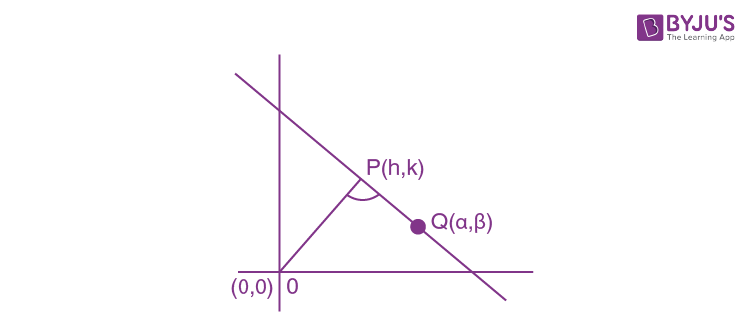$$\left ( \frac{k-\beta }{h-\alpha } \right )\left ( \frac{k}{h} \right )=-1$$

⇒k2 – kβ = – (h2 –hα)

⇒ h2 + k2 –hα– kβ = 0

⇒Required locus is x2 + y2 –α x –β y = 0

Question 45: If the point of intersection of the lines 2ax + 4ay + c = 0 and 7bx + 3by – d = 0 lies in the 4th quadrant and is equidistant from the two axes, where a, b, c and d are non-zero numbers, then ad: bc equals to

1. a. 2 : 3
2. b. 2 : 1
3. c. 1 : 1
4. d. 3 : 2

Solution:

Let point on 4th quadrant which is equidistant from both the axis is (α, –α)

⇒ L1: 2aα – 4a α + c = 0 ⇒α=c/2a …………(1)

⇒L2: 7bα – 3bα – d = 0⇒ α = d/4b …………..(2)

From equation (1) & (2)

⇒ (c/2a) = (d/4b)

⇒ ad : bc = 2 : 1

Question 46: A variable circle passes through the fixed point A(p, q) and touches x-axis. The locus of the other end of the diameter through A is

1. a. (x – p)2 = 4qy
2. b. (x – q)2 = 4py
3. c. (y – p)2 = 4qx
4. d. (y – q)2 = 4px

Solution:

Let other end is (h, k) then centre equal to

$$\left ( \frac{p+h}{2},\frac{q+k}{2} \right )$$

Because circle touches x-axis hence radius =

$$\left | \frac{q+k}{2} \right |$$

We know

⇒ (AB) Diameter = 2r

$$\sqrt{(h-P)^{2}+(k-q)^{2}}=2\left | \frac{q+k}{2} \right |$$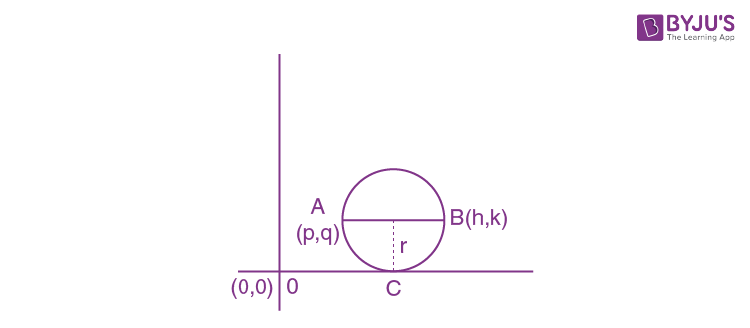On squaring both sides

⇒ (h – p)2 + (k – q)2 = (q + k)2

⇒ (h – p)2 = (k + q)2 – (k – q)2

⇒ Required locus is = (x – p)2 = 4qy

Question 47: If P(0, 0), Q(1, 0) and R

$$\left [ \frac{1}{2},\frac{\sqrt{3}}{2} \right ]$$
are three given points, then the centre of the circle for which the lines PQ, QR and RP are the tangents is

1. a.
$$\left [ \frac{1}{2},\frac{1}{4} \right ]$$
2. b.
$$\left [ \frac{1}{2},\frac{\sqrt{3}}{4} \right ]$$
3. c.
$$\left [ \frac{1}{2},\frac{1}{2\sqrt{3}} \right ]$$
4. d.
$$\left [ \frac{1}{2},\frac{-1}{\sqrt{3}} \right ]$$

Solution:

ΔPQR is equilateral triangle

So, incentre is same as centroid

⇒ incentre = centroid = centre of circle =

$$\left [ \frac{1+\frac{1}{2}+0}{3},\frac{0+0+\frac{\sqrt{3}}{2}}{3} \right ]=\left [ \frac{1}{2},\frac{1}{2\sqrt{3}}, \right ]$$

Question 48: For the hyperbola

$$\frac{x^{2}}{cos^{2}\alpha }-\frac{y^{2}}{sin^{2}\alpha }=1$$
, which of the following remains fixed when α varies?

1. a. Directrix
2. b. Vertices
3. c. foci
4. d. Eccentricity

Solution:

Focus are =

$$(\pm \sqrt{a^{2}+b^{2},0})=(\pm \sqrt{cos^{2}\alpha +sin^{2}\alpha },0)$$

⇒ focus are (±1,0), which is independent of α

⇒focus are fixed

Question 49: S and T are the foci of an ellipse and B is the end point of the minor axis. If STB is equilateral triangle, the eccentricity of the ellipse is

1. a. 1/4
2. b. 1/3
3. c. 1/2
4. d. 2/3

Solution:

S = (ae, 0)

T = (–ae, 0)

B =(0,b)

⇒ (ST)2 = (SB)2

⇒ (2ae)2 = (ae)2 + b2

⇒3(ae)2 = b2

⇒b2 = 3(a2 – b2)

$$\frac{b^{2}}{a^{2}}=\frac{3}{4}$$

$$e=\sqrt{1-\frac{b^{2}}{a^{2}}}$$

$$e=\sqrt{1-\frac{3}{4}}$$

e=1/2

Question 50: The equation of the directrices of the hyperbola 3x2 – 3y2 – 18x + 12y + 2 = 0 is

1. a.
$$x=3\pm \sqrt{\frac{13}{6}}$$
2. b.
$$x=3\pm \sqrt{\frac{6}{13}}$$
3. c.
$$x=6\pm \sqrt{\frac{13}{3}}$$
4. d.
$$x=6\pm \sqrt{\frac{3}{13}}$$

Solution:

Equation of hyperbola is 3(x2 – 6x) – 3 (y2 –4y) + 2 = 0

⇒ (x – 3)2 – (y – 2)2 = 13/3

$$\Rightarrow \frac{(x-3)^{2}}{\sqrt{\frac{13}{3}}}-\frac{(y-2)^{2}}{\sqrt{\frac{13}{3}}}=1\Rightarrow e=\sqrt{2}$$

We know equation of directrix is X =

$$\pm \frac{a}{e}$$

$$x-3=\pm \frac{\sqrt{13/3}}{\sqrt{2}}$$

$$x=3\pm \frac{\sqrt{13/3}}{\sqrt{2}}$$

Question 51: The graphs of the polynomial x2 – 1 and cos x intersect

1. a. at exactly two points
2. b. at exactly 3 points
3. c. at least 4 but at finitely many points
4. d. at infinitely many points

Solution:

y = x2 – 1 & y = cos x

intersect at exactly two points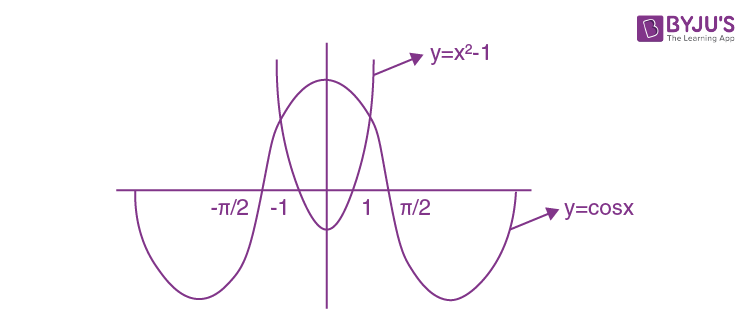Question 52: A point is in motion along a hyperbola y = 10/x so that its abscissa x increases uniformly at a rate of 1 unit per second. Then, the rate of change of its ordinate, when the point passes through (5, 2)

1. a. increases at the rate of 1/2 unit per second
2. b. decreases at the rate of 1/2 unit per second
3. c. decreases at the rate of 2/5 unit per second
4. d. increases at the rate of 2/5 unit per second

Solution:

Given

(dx/dt) = 1 unit per second

y = 10/x ………(1)

differentiating with respect to x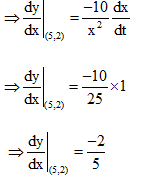⇒Ordinate decreases at rate unit per second.

Question 53: Let a = min{x2 + 2x + 3: x

$$\epsilon$$
R} and b =
$$\lim_{x\rightarrow 0}\frac{1-cos\theta }{\theta ^{2}}$$
. Then
$$\sum_{r=0}^{n}a^{r}b^{n-r}$$
is

1. a.
$$\frac{2^{n+1}-1}{3.2^{n}}$$
2. b.
$$\frac{2^{n+1}+1}{3.2^{n}}$$
3. c.
$$\frac{4^{n+1}-1}{3.2^{n}}$$
4. d. (1/2)(2n – 1)

Solution:

a = min [x2 + 2x + 3; x

$$\epsilon$$
R]

a = min [(x+1)2+2]

⇒ a = 2

$$b=\lim_{\theta \rightarrow 0}\frac{1-cos\theta }{\theta ^{2}}=\frac{1}{2^{2}}.\frac{2sin^{2}\frac{\theta }{2}}{\frac{\theta ^{2}}{2^{2}}}=\frac{1}{2}$$

⇒b=1/2

Now,

$$\sum_{r=0}^{n}a^{r}b^{n-r}=a^{0}b^{n}+ab^{n-1}+a^{2}b^{n-2}+.........+a^{n}b^{0}$$

$$=\left ( \frac{1}{2} \right )^{n}+\frac{2}{2^{n-1}}+\frac{2^{2}}{2^{n-2}}+.........+2^{n}$$

$$=\left ( \frac{1}{2} \right )^{n}\left [ 1+4+4^{2}+.......+4^{n} \right ]$$

$$\frac{1}{2^{n}}\left [ \frac{4^{n+1}-1}{4-1} \right ]$$

$$\left [ \frac{4^{n+1}-1}{3.2^{n}} \right ]$$

Question 54: Let a > b >0 and I(n) = a1/n – b1/n, J(n) = ((a – b)1/n for all n ≥ 2. then

1. a. I(n) < J(n)
2. b. I(n) > J(n)
3. c. I(n) = J(n)
4. d. I(n) + J(n) = 0

Solution:

Given

⇒a > b > 0

⇒ a > b

⇒ (b/a) <1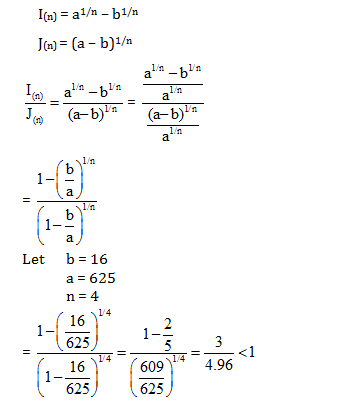= I(n) < J(n)

Question 55: Let

$$\hat{\alpha },\hat{\beta },\hat{\gamma }$$
be three unit vectors such that
$$\hat{\alpha }\times (\hat{\beta }\times \hat{\gamma })=\frac{1}{2}(\hat{\beta }\times \hat{\gamma })$$
where
$$\hat{\alpha }\times (\hat{\beta }\times \hat{\gamma })=(\hat{\alpha }.\hat{\gamma })\hat{\beta }-(\hat{\alpha }.\hat{\beta })\hat{\gamma }$$
.If
$$\hat{\beta }$$
is not parallel to , then the angle between α and β is

1. a. 5π/6
2. b. π/6
3. c. π/3
4. d. 2π/3

Solution:

$$\left |\hat{\alpha } \right |= \left | \hat{\beta } \right |=\left | \hat{\gamma }\right |=1 (given)$$

Now,

$$\hat{\alpha }\times (\hat{\beta }\times \hat{\gamma })=\frac{1}{2}(\hat{\beta }\times \hat{\gamma })$$

On comparing both sides

$$\Rightarrow -\hat{\alpha }.\hat{\beta }=\frac{1}{2}$$

$$\Rightarrow \hat{\alpha }.\hat{\beta }=\frac{-1}{2}$$

$$\left | \hat{\alpha } \right | .\left | \hat{\beta } \right |cos\theta =\frac{-1}{2}$$

$$\theta =\frac{2\pi }{3}$$

Question 56: The position vectors of the points A, B, C and D are

$$3\hat{i}-2\hat{j}-\hat{k}$$
,
$$2\hat{i}-3\hat{j}+2\hat{k}$$
,
$$5\hat{i}-\hat{j}+2\hat{k}$$
and
$$4\hat{i}-\hat{j}+\lambda \hat{k}$$
respectively. If the points A, B, C and D lie on a plane, the value of λ is

1. a. 0
2. b. 1
3. c. 2
4. d. – 4

Solution:

Given

A, B, C, & D are on a plane

Since,

$$\vec{AB}, \vec{AC},\vec{AD}$$
are coplanar

Since,

$$[\vec{AB},\vec{AC},\vec{AD}]=0$$

$$\Rightarrow \begin{vmatrix} 1 & 1 & -3\\ -2& -1 &-3 \\ -1& -1 & -1-\lambda \end{vmatrix}=0$$

$$R_{3}\rightarrow R_{3}+R_{1}$$

$$\Rightarrow \begin{vmatrix} 1 & 1 & -3\\ -2& -1 &-3 \\ 0& 0 & -4-\lambda \end{vmatrix}=0$$

⇒ (-4-λ) (-1 + 2) = 0

⇒λ = -4

Question 57: A particle starts at the origin and moves 1 unit horizontally to the right and reaches P1, then it moves ½ unit vertically up and reaches P2, then it moves 1/4 unit horizontally to right and reaches P3, then it moves 1/8 unit vertically down and reaches P4, then it moves 1/16 unit horizontally to right and reaches P5 and so on. Let Pn = (xn, yn) and xn =α and yn = β. Then (α,β) is

1. a. (2, 3)
2. b.
$$\left [ \frac{4}{3},\frac{2}{5} \right ]$$
3. c.
$$\left [ \frac{2}{5},1 \right ]$$
4. d.
$$\left [ \frac{4}{3},3 \right ]$$

Solution: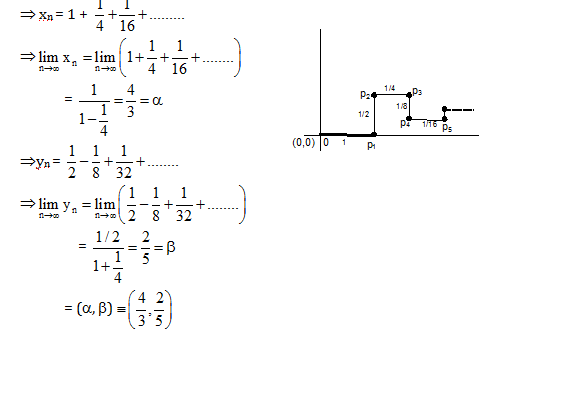Question 58: For any non-zero complex number z, the minimum value of |z| + |z – 1| is

1. a. 1
2. b. 1/2
3. c. 0
4. d. 3/2

Solution:

Using inequality |A| + |B|≥ |A – B |

⇒|z| +|z – 1|≥ |z –(z – 1)|

⇒ |z| + |z–1| ≥ 1

⇒ minimum value of |z| + |z – 1| is 1

Question 59: The system of equations

λx + y + 3z = 0

2x + µy – z = 0

5x + 7y + z = 0

Has infinitely many solutions in R. Then,

1. a. λ = 2, µ = 3
2. b. λ = 1, µ = 2
3. c. λ = 1, µ = 3
4. d. λ = 3, µ = 1

Solution:

For infinitely many solution Δ= 0

$$\Rightarrow\begin{vmatrix} \lambda &1 &3 \\ 2 & \mu & -1\\ 5& 7 &1 \end{vmatrix}=0$$

λ μ + 7 λ –7 + 42 – 15 μ =0

⇒ (λ – 15) (μ + 7)+ 140 =0

Now check from options

⇒ (λ, μ) = (1, 3)

Question 60: Let f: X

$$\rightarrow$$
Y and A, B are non-void subsets of Y, then (where the symbols have their usual interpretation)

1. a. f–1(A) – f–1(B)
$$\supset$$
f–1(A – B) but the opposite does not hold
2. b. f–1(A) – f–1(B)
$$\subset$$
f–1(A – B) but the opposite does not hold
3. c. f–1(A – B) = f–1(A) – f–1(B)
4. d. f–1(A – B) = f–1(A)
$$\cup$$
f–1(B)

Solution:

We can see that pre-image of A – B i.e. f–1(A – B) will be f–1(A) – f–1(B)

Therefore, f–1 (A – B) = f–1(A) – f–1(B)Question 61: Let S, T, U be three non-void sets and f : S

$$\rightarrow$$
T, g : T
$$\rightarrow$$
U be so that gof : S
$$\rightarrow$$
U is surjective. Then

1. a. g and f are both surjective
2. b. g is surjective, f may not be so
3. c. f is surjective, g may not be so
4. d. f and g both may not be surjective

Solution:

Obvious g is surjective otherwise gof cannot be surjective but there is no need of “f” to be surjective.

Example.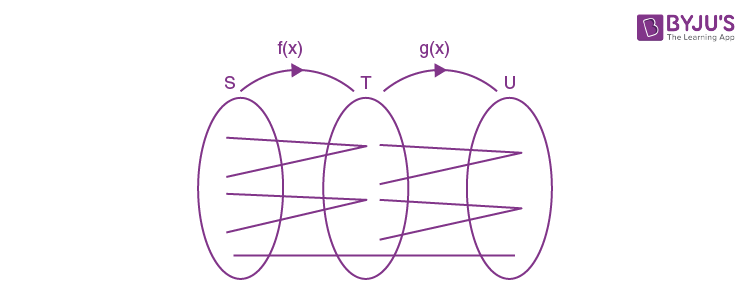Hence f(x) is not surjective still gof is surjective

Question 62: The polar coordinate of a point P is

$$\left [ 2,\frac{-\pi }{4} \right ]$$
. The polar coordinate of the point Q, which is such that the line joining PQ is bisected perpendicularly by the initial line, is

1. a.
$$\left [ 2,\frac{\pi }{4} \right ]$$
2. b.
$$\left [ 2,\frac{\pi }{6} \right ]$$
3. c.
$$\left [ -2,\frac{\pi }{4} \right ]$$
4. d.
$$\left [ -2,\frac{\pi }{6} \right ]$$

Solution:

line joining PQ is bisected by x –axis

So point Q is

$$\left [ 2,\frac{\pi }{4} \right ]$$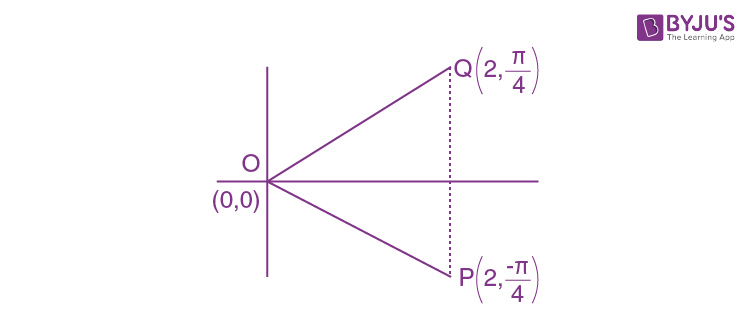Question 63: The length of conjugate axis of a hyperbola is greater than the length of transverse axis. Then the eccentricity e is

1. a. = √2
2. b. > √2
3. c. < √2
4. d.1/√2

Solution:

b > a (given)

On squaring both sides

$$\Rightarrow \frac{b^{2}}{a^{2}}> 1$$

On adding 1 in both sides

$$1+\frac{b^{2}}{a^{2}}> 2$$

Taking root both sides

$$\Rightarrow \sqrt{1+\frac{b^{2}}{a^{2}}}> \sqrt{2}$$

$$\Rightarrow e> \sqrt{2}$$

Question 64: The value of is

$$\lim_{x\rightarrow 0}\frac{x}{p}\left [ \frac{q}{x} \right ]$$

1. a.
$$\frac{\left [ q \right ]}{p}$$
2. b. 0
3. c. 1
4. d. ∞

Solution: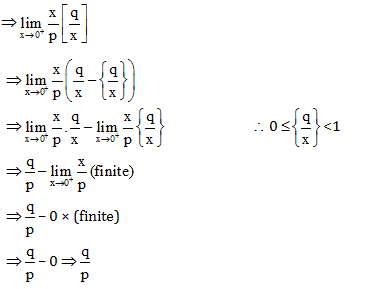Question 65: Let f(x) = x4 – 4x3 + 4x2 + c, c

$$\epsilon$$
R. Then

1. a. f(x) has infinitely many zeroes in (1, 2) for all c
2. b. f(x) has exactly one zero in (1, 2) if –1 < c < 0
3. c. f(x) has double zeroes in (1, 2) if –1 < c < 0
4. d. whatever be the value of c, f(x) has no zero in (1, 2)

Solution:

f(x) = x4 – 4x3 + 4x2 + c,

Using IVT theorem

For atleast one root/zero = f(1) . f(2) < 0

= (1 + c) c< 0

= c

$$\epsilon$$
(–1, 0)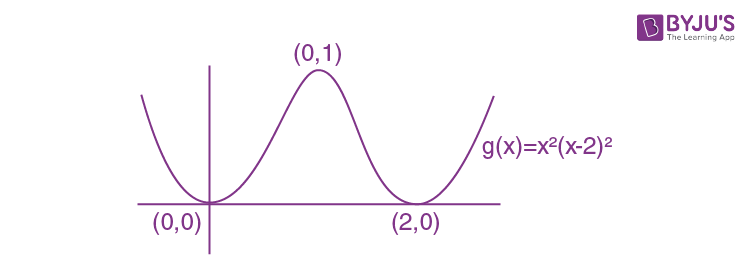f(x) = x2(x – 2)2 + c

⇒f(x) has exactly one zero in (1, 2) if (–1, 0)

Question 66: Let f and g be differentiable on the interval I and let a, b

$$\epsilon$$
I, a < b. Then

1. a. If f(a) = 0 = f(b), the equation f'(x) + f(x)g'(x) = 0 is solvable in (a, b)
2. b. If f(a) = 0 = f(b), the equation f'(x) + f(x)g'(x) = 0 may not be solvable in (a, b)
3. c. If g(a) = 0 = g(b), the equation g'(x) + kg(x) = 0 is solvable in (a, b), k
$$\epsilon$$
R
4. d. If g(a) = 0 = g(b), the equation g'(x) + kg(x) = 0 may not be solvable in (a, b), k
$$\epsilon$$
R

Solution:

f(a) = 0 = f(b) ⇒f’(a)f’ (b) < 0

Let h(x) = f’ (x) + f(x) g’ (x) …………..(1)

Put x = a in equation (1)

h(a) = f’(a) since f(a) = 0

Put x = b in equation (1)

h(b) = f’ (b) since f(b) = 0

Therefore, h(a) . h(b) < 0 ⇒ h(x) = 0 has roots between (a, b)

Similarly, g’ (x) + kg(x) = 0 has roots between (a, b) as g(a) = 0 = g(b)

Question 67: Consider the function f(x) = (x3/4) – sin πx + 3

1. a. f(x) does not attain value within the interval [–2, 2]
2. b. f(x) takes on the value 2 in the interval [–2, 2]
3. c. f(x) takes on the value 3 in the interval [–2, 2]
4. d. f(x) takes no value p, 1 < p < 5 in the interval [–2, 2]

Solution:

$$f(x)=\frac{x^{3}}{4}-sin\pi x+3$$

f(–2) = 1

f(2) = 5

since function is continuous

By intermediate value theorem, f(x) takes all values between 1 to 5

Question 68:Let

$$I_{n}=\int_{0}^{1}x^{n}tan^{-1}xdx$$
. If anIn+2 + bnIn = cn for all n ≥ 1, then

1. a. a1, a2, a3 are in G.P.
2. b. b1, b2, b3 are in A.P.
3. c. c1, c2, c3 are in H.P.
4. d. a1, a2, a3 are in A.P

Solution:

$$I_{n}=\int_{0}^{1}x^{n}tan^{-1}xdx$$

Using Integration by parts

$$\Rightarrow {I}_{n}=\left.\tan ^{-1} {x} \frac{{x}^{{n}+1}}{n+1}\right|_{0} ^{1}-\int_{0}^{1} \frac{{x}^{n+1}}{n+1} \cdot\left(\frac{1}{1+{x}^{2}}\right) dx$$

$$\Rightarrow(n+1) I_{n}=\frac{\pi}{4}-\int_{0}^{1} \frac{x^{n+1}}{1+x^{2}} d x$$
----------(1)

Put n → n + 2 in equation (1)

$$\Rightarrow(n+3) I_{n+2}=\frac{\pi}{4}-\int_{0}^{1} \frac{x^{n+3}}{1+x^{2}} d x$$
--------(2)

From equation(1) + (2)

⇒ (n +1)In + (n + 3) In+2 =

$$\frac{\pi }{2}-\frac{1}{n+2}$$

⇒ on comparing with given equation

⇒an = n + 1, bn = n+ 3,

$$c_{n}=\frac{\pi }{2}-\frac{1}{n+2}$$

Question 69: Two particles A and B move from rest along a straight line with constant accelerations f and h respectively. If A takes m seconds more than B and describes n units more than that of B acquiring the same speed, then

1. a. (f + h)m2 = fhn
2. b. (f – fh)m2 = fhn
3. c. (h – f)n = fhm2
4. d. (1/2)(f + h)n =fhm2

Solution:

S + n = (1/2) f(t + m2)and S =(1/2)ht2 , V = ht

(1/2)ht2 +n= (1/2) f(t + m2) ……….(1)

Also V = 0 + ht = 0 + f(t +m) ⇒ t + m =(ht/f) (put in equation (1))

from equation (1)

$$\Rightarrow \frac{1}{2} \mathrm{ht}^{2}+\mathrm{n}=\frac{1}{2} \mathrm{f}\left(\frac{\mathrm{ht}}{\mathrm{f}}\right)^{2}$$

$$\Rightarrow \mathrm{t}^{2}=\frac{2 \mathrm{nf}}{\mathrm{h}(\mathrm{h}-\mathrm{f})}$$

Also,

$$\mathrm{ht}=\mathrm{f}(\mathrm{t}+\mathrm{m}) \Rightarrow \mathrm{t}^{2}=\frac{\mathrm{m}^{2} \mathrm{f}^{2}}{(\mathrm{h}-\mathrm{f})^{2}}$$

Therefore,

$$\frac{2 \mathrm{nf}}{\mathrm{h}(\mathrm{h}-\mathrm{f})}=\frac{\mathrm{m}^{2} \mathrm{f}^{2}}{(\mathrm{h}-\mathrm{f})^{2}}$$

$$\Rightarrow \mathrm{n}(\mathrm{h}-\mathrm{f})=\mid \frac{1}{2} \mathrm{f} \mathrm{hm}^{2}$$

Question 70: The area bounded by y = x + 1 and y = cos x and the x-axis, is

1. a. 1 sq. unit
2. b. (3/2) sq. unit
3. c. (1/4) sq. unit
4. d. (1/8) sq. unit

Solution: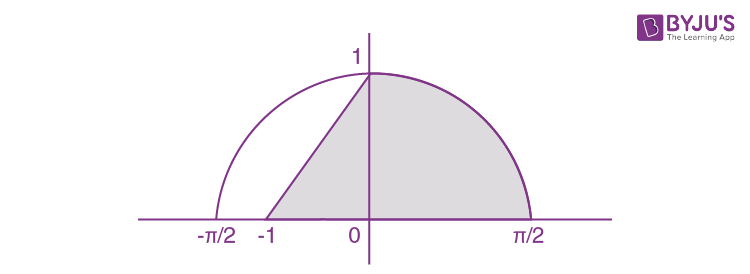$$Area=\frac{1}{2}\times 1\times 1+\int_{0}^{\pi /2}cosxdx$$

$$=\frac{1}{2}+\left.\sin x\right|_{0} ^{\pi / 2}$$

= (1/2)+1= 3/2

Question 71: Let x1, x2 be the roots of x2 – 3x + a = 0 and x3, x4 be the roots of x2 – 12x + b = 0. If x1< x2< x3< x4 and x1, x2, x3, x4 are in G.P. then ab equals

1. a. 24/5
2. b. 64
3. c. 16
4. d. 8

Solution: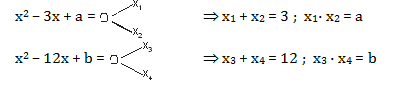Since x1 , x2, x3, x4 are in G.P, then

$$\frac{x_{3}+x_{4}}{x_{1}+x_{2}}=\frac{12}{3}$$

$$\frac{Ar^{2}+Ar^{3}}{A+Ar}=4$$

⇒r2 = 4 ⇒ x = 2 (since G.P is increasing)

⇒x1 + x2 = 3

⇒A + Ar = 3

⇒ A(3) = 3

⇒A = 1

Therefore, ab = x1 • x2 • x3 • x4 = 1.2. 4.8 = 64

Question 72: If q

$$\epsilon$$
R and
$$\frac{1-icos\theta }{1+2icos\theta }$$
is real number, then θ will be (when I : Set of integers)

1. a. (2n + 1) (π/2) , n
$$\epsilon I$$
2. b.3nπ/2 , n
$$\epsilon$$
I
3. c. nπ, n
$$\epsilon$$
I
4. d. 2nπ, n
$$\epsilon$$
I

Solution:

Using rationalization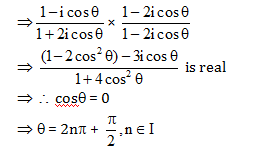Question 73: Let A =

$$\begin{pmatrix} 3 & 0 &3 \\ 0& 3 &0 \\ 3& 0 & 3 \end{pmatrix}$$
. Then the roots of the equation (A – λI3) = 0

1. a. 3, 0, 3
2. b. 0, 3, 6
3. c. 1, 0, –6
4. d. 3, 3, 6

Solution:

(A – λI3) = 0

$$\begin{pmatrix} 3-\lambda & 0 &3 \\ 0& 3-\lambda &0 \\ 3& 0 & 3-\lambda \end{pmatrix}$$

⇒ (3 – λ)3 – 9 (3 – λ) = 0

⇒ (3 – λ) [(3 – λ)2 – 32 = 0

⇒3 – λ = 0 or 3 – λ – 3 = 0 or 3 – λ +3 =0

⇒λ = 0, 3, 6

Question 74: Straight lines x – y = 7 and x + 4y = 2 intersect at B. Points A and C are so chosen on these two lines such that AB = AC. The equation of line AC passing through (2, –7) is:

1. a. x – y – 9 = 0
2. b. 23x + 7y + 3 = 0
3. c. 2x – y – 11 = 0
4. d. 7x – 6y – 56 = 0

Solution:

AB = AC (given)

∠ABC = ∠BCA

Let slope of AC is m.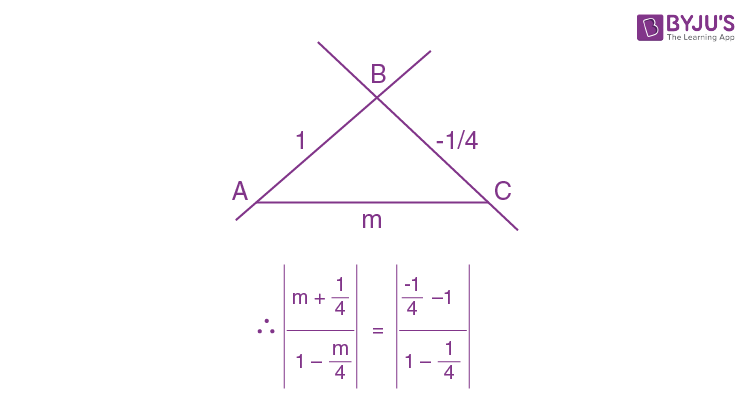m = -23/7, 1 (rejected)

Therefore, equation of line is 23x + 7y + 3 = 0

Question 75: Equation of a tangent to the hyperbola 5x2 – y2 = 5 and which passes through an external point (2, 8) is

1. a. 3x – y + 2 = 0
2. b. 3x + y – 14 = 0
3. c. 23x – 3y – 22 = 0
4. d. 3x – 23y + 178 = 0

Solution:

$$\frac{x^{2}}{1}-\frac{y^{2}}{5}=1$$

Let the tangent of hyperbola

$$y=mx\pm \sqrt{a^{2}m^{2}-b^{2}}$$

$$y=mx\pm \sqrt{m^{2}-5}$$
……(1)

Passes through (2, 8)

⇒ (8 – 2m) =

$$\pm \sqrt{m^{2}-5}$$

On squaring both sides

⇒ (8 – 2m)2 = (m2 – 5)

⇒m = 3 or (23/3) (put in equation (1))

So equation of tangent is ⇒

$$y=3x\pm 2$$
or
$$y=\frac{23x}{3}\pm \frac{22}{3}$$

⇒ y = 3x + 2, y = 3x – 2, 3y = 23x + 22, 3 y = 23x – 22

### WBJEE 2019 Maths Question Paper with Solutions